GFG App
Open AppBrowser
Continue

# Class 10 NCERT Solutions- Chapter 6 Triangles – Exercise 6.5 | Set 1

### (iv) 13 cm, 12 cm, 5 cm

Solution:

(i) Given: 7cm, 24cm, 25cm

(25)2= 25 * 25 = 625

∴They are sides of a right ∆ and hypotenuse = 625

(ii) Given: 3cm, 8cm, 6cm

(8)2 = 8 * 8 = 64

They are not side of right triangle.

(iii) Given: 50cm, 80cm, 100cm

(100)2 = 100 * 100 = 10000

(50)2 + (80)2 = 2500 + 6400

Therefore, this is not right triangle.

(iv) Given: 13cm, 12cm, 5cm

(13)2 = 13 * 13 = 169

(12)2 + (5)2 = 169

Therefore, this is the sides of right triangle and hypotenuse = 169

### Question 2. PQR is a triangle right-angled at P and M is a point on QR such that PM ⊥ QR. Show that PM2 = QM × MR.

Solution:

Given: A right ∆PQR right-angled at P and PM⊥QR

To show: PM2 = QM * MR

PM * PM = QM * MR

In ∆PMQ and ∆PMR

∠1 =∠2           -(each 90°)

∠1 +∠2 +∠3 = 180°

90° + ∠4 + ∠5 = 180°

∠4 +∠5 = 180° – 90°

∠3 + ∠4 = 180° – 90°

∠4 +∠5 = ∠3 +∠4

∴∠5 =∠3

∴∆PMQ ~ ∆RMPMP2 = MQ.PM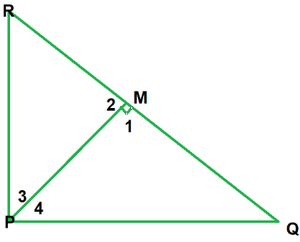### (iii) AD2 = BD × CD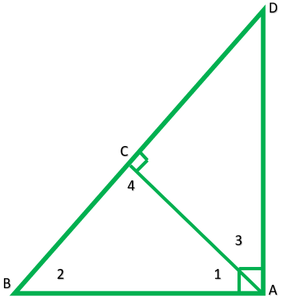Solution:

Given: A right ∆ABD, right angled at A and AC⊥ BD

To show:

(i)AB2 = BC × BD

(ii) AC2 = BC × DC

(iii) AD2 = BD × CD

(i) AB2 = BC × BD

AB * AB = BC.BD

In ∆ ABC and ∆ABD

∠B =∠B          -(common)

∠BCA = ∠A          -(each 90°)

∴ ∆ABC~∆DBAAB2 = BC * DC

(ii) AC2 = BC * DC

AC * AC = BC * DC

In ∆ABC and ∆ACD

∠ACB = ∠ACD          -(each 90°)

In ∆ ACB

∠1 + ∠2 + ∠3 = 180°

∠1 + ∠2 + 90° = 180°

∠1 + ∠2 = 90°           -(1)

∠1 + ∠3 = 90°          -(2)

∠1 + ∠2 =∠1 + ∠3

∴ ∠2 = ∠3

∴ ∆ABC~∆DACAC2 = BC * CD

(iii) AD2 = BD × CD

In ∆ABD and ∆ACD

∠A =∠ACD          -(each 90)

∠D =∠D          -(common)### Question 4. ABC is an isosceles triangle right angled at C. Prove that AB2 = 2AC2.

Solution:

Given: ∆ABC right angled at B

To prove: AB2 = 2AC2

According to Pythagoras theorem,

(AB)2 = (AC)2 + (BC)2

(AB)2 = (AC)2 + (AC)2           -(BC = AC)

AB2 = 2AC2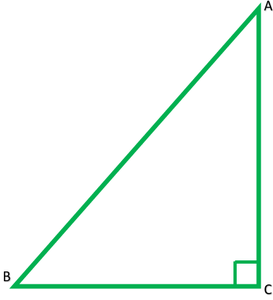### Question 5. ABC is an isosceles triangle with AC = BC. If AB2 = 2AC2, prove that ABC is a right triangle.

Solution:

Given: ∆ABC is an isosceles triangle in which

AC = BC

AB2 = 2AC2

To prove: ABCD is right ∆

AB2 = 2AC2

AB2 = AC2 + AC2

∴ By the, converse of Pythagoras theorem ∆ACB is a right ∆ at C.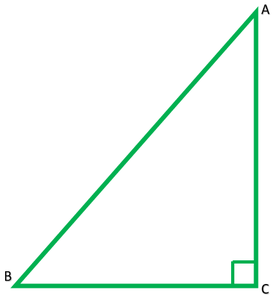### Question 6. ABC is an equilateral triangle of side 2a. Find each of its altitudes.

Solution:

Let ABC is an equilateral triangle of each 2a units

3a2 = (AB)2

√3 a2 = AD               ∴ Each of its altitude= √3 a unit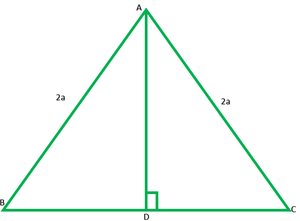### Question 7. Prove that the sum of the squares of the sides of the rhombus is equal to the sum of the squares of its diagonals.

Solution:

Given: ABCD is a rhombus

To prove: AB2 + BC2 + CD2 + DA2

Proof: ABCD is rhombus and let diagonals AC and BD bisect each other at O.

∴ ∠AOB =∠BOC =∠COD =∠DOA = 90°

In ∆AOB

AB2 = AC2 + BO2

AB2 = (1/2AC2) + (1/2BD2)

AB2 = 1/4(AC2 + BD2)

4AB2 = AC2 + BD2          -(1)

Similarly, in ∆BOC

4BC2 = AC2 + BD2          -(2)

4CD2 = AC2 + BD2          -(3)

4AD2 = AC2 + BD2          -(4)

Adding 1, 2, 3, and 4

4AB2 + 4BC2 + 4CD2 + 4DA2 = 4(AC2 + BD2)

4(AB2 + BC2 + CD2 + DA2) = 4(AC2 + BD2)

∴ AB2 + BC2 + CD2 + DA2 = AC2 + BD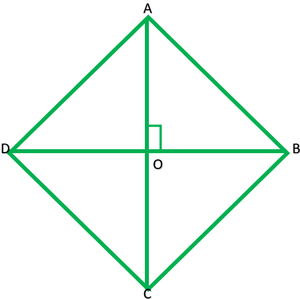### (ii) AF2 + BD2 + CE2 = AE2 + CD2 + BF2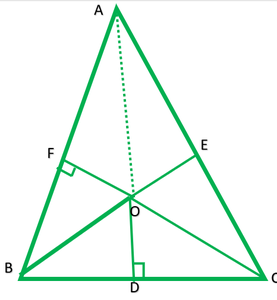Solution:

(i) In right ∆AFO, by Pythagoras theorem

OA2 = AP2 + OF2          -(1)

In right ∆ODB, by Pythagoras theorem

OB2 = BD2 + OD2          -(2)

In right ∆OEC, by Pythagoras theorem

OC2 = CE2 + OE2          -(3)

OA2 + OB2 + OC2 = AF2 + BD2 + CF2 + OD2 + OE2

OA2 + OB2 + OC2 – OE2 – OD2 – OE2 = AF2 + BD2 + CE2

(ii) AF2 + BD2 + CE2 = AE2 + CD2 + BF2

OB2 = BD2 + OD2          -(1)

OC2 = DC2 + OD2          -(2)

Subtracting (1) from (2) we get,

OB2 – OC2 = BD2 + OD2 – (DC2 + OD2)

= BD2 + OD2 – DC2 – OD2

= BD2 – DC2          -(3)

Similarly,

OC2 – AD2 = CE2 – EA2          -(4)

AO2 = BO2 = AF2 – FB2          -(5)

Adding 3, 4, and 5, we get,

OB2 – OC2 + OC2 – AO2 + AO2 – BO2 = BD2 – DC2 + CE2 – EA2 + AF2 – FB2

0 + DC2 + EA2 + FB2 = BD2 + CD2 + AF2

### Question 9. A ladder 10 m long reaches a window 8 m above the ground. Find the distance of the foot of the ladder from base of the wall.

Solution:

AB is wall  = 8m

BC = ?

In right ∆ABC, by Pythagoras theorem

(AC)2 = (AB)2 + (BC)2

(10)2 = (8)2 + (BC)2

100 = 64 + (BC)2

100 – 64 = (BC)2

36 = (BC)2

√36 = BC

√(6 * 6) = BC

6 = BC

Hence, the foot of the ladder is at a distance of 6 m from the base of the wall.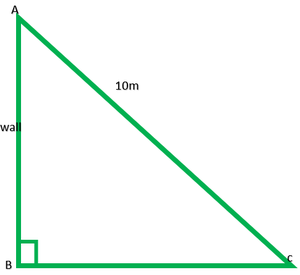### Question 10. A guy wire attached to a vertical pole of height 18 m is 24 m long and has a stake attached to the other end. How far from the base of the pole should the stake be driven so that the wire will be taut?

Solution:

In fig.

AB is pole = 18m

AC is wire = 24m

BC = ?

In right ∆ABC, by Pythagoras theorem

(AC)2 = (AB)2 + (BC)2

(24)2 = (18)2 + (BC)2

576 = 324 + (BC)2

576 – 324 = (BC)2

252 = (BC)2

√252 = BC

√(2 * 2 * 3 * 3 * 7) = BC

2 * 3√7 = BC

6√7 = BC

∴ Hence, the stake may be placed at distance of 6√7 from the base of the pole.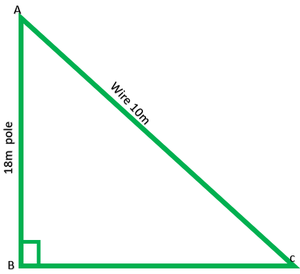### Chapter 6 Triangles – Exercise 6.5 | Set 2

My Personal Notes arrow_drop_up